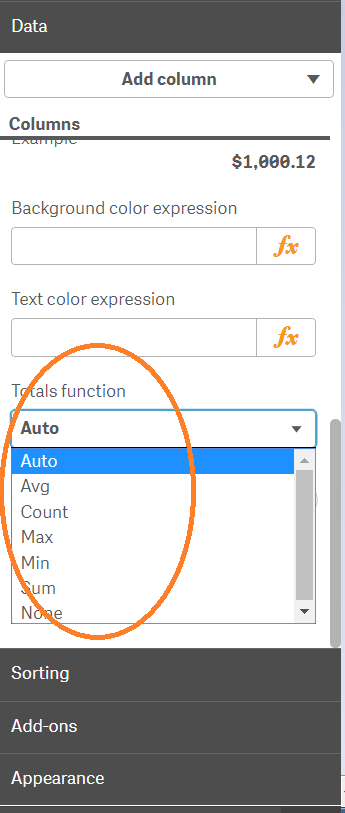New to Qlik Sense

Discussion board where members can get started with Qlik Sense.

Announcements
QlikWorld 2020: Join us May 11 - 14, 2020 in Phoenix, AZ. Register early and save \$400. Learn More
Contributor

Totals function straight table

What does the 'Auto' aggregation perform for the totals function? It's not average/mean; I tried that and the total was different. I tried the other total functions ie max, min, count etc. and 'Auto' was not actually any.  I could not find specific information about this in the docs for this as it only says, "Select which aggregation function you want to use for the Totals row in the table. You set the position of the Totals row under Presentation".1 Solution

Accepted SolutionsMVP & Luminary

Re: Totals function straight table

I don't know how qlik sense these totals handled (I don't work much with sense) and I don't use these features within qlikview. By simple expressions like: sum(value) or count(item) the totals are always working like expected. By an quote expression like: sum(value) / count(item) will be summed and counted on the total-level without any considerations of the used dimensions and those results are than divided - and this is often not the wanted result and therefore the usage of the aggr-function to define exactly how the totals should be calculated.

- Marcus

7 RepliesMVP & Luminary

Re: Totals function straight table

Try it without these auto-totals and an expression like:

avg(aggr(YourExpression, YourDimensions))

- Marcus

Esteemed Contributor II

Re: Totals function straight table

Can you a sample app? or screenshots ?? Sorry but your description is a bit confusing

I don't know what you mean by "Auto" aggregation  and in the end you are referring to expression totals!

Contributor

Re: Totals function straight table

I tried it using avg(aggr(YourExpression, YourDimensions)) within the table. In this case 'Auto' was the exact same as Avg. Why does this 'Avg' == 'Auto' but the normal summation 'Avg' (did not)= 'Auto'? Can you connect the dots for me?

Contributor

Re: Totals function straight table

Ok, thanks. I updated my post with an screenshot.MVP & Luminary

Re: Totals function straight table

I don't know how qlik sense these totals handled (I don't work much with sense) and I don't use these features within qlikview. By simple expressions like: sum(value) or count(item) the totals are always working like expected. By an quote expression like: sum(value) / count(item) will be summed and counted on the total-level without any considerations of the used dimensions and those results are than divided - and this is often not the wanted result and therefore the usage of the aggr-function to define exactly how the totals should be calculated.

- Marcus

Contributor

Re: Totals function straight table

I think you're right. Maybe, like you said, it tries to repeat the existing aggregate functions that are already present in the expression.

New Contributor

Re: Totals function straight table

I'm having the same issue --

I am trying to calculate the avg days between two timestamps. It seems that line by line my expression is working correctly, but when it's in a table format and totaled the "Totals Function" when set to Auto does not give me the correct value. When I force the totals function to Avg it then does give me the correct value. Has anyone figured out why this happens? Why is the Auto function not = to Avg?

my "1. Open Job" expression is =           avg(timestamp#([First Approved]) - timestamp#([First Draft]))

It is 1.53 total when set to Avg, but is giving me 1.45 when set to Auto???? In my KPIs on another page it's displaying 1.45.

My first hunch was it had something to do with the format being timestamp? Please help!